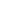#Introduction to Fractions MTH-1004 is a Course

## Introduction to Fractions MTH-1004

Self-paced

### Full course description

Course Description

A fraction represents part of a whole. Every fraction has a numerator and denominator. In this course, you’ll learn what a fraction is and the parts that make one up. You’ll find out how to compare fractions. You’ll also discover techniques for finding the least common denominator and ordering fractions.

By the end of this course, you will be able to

• Understand the importance of equal parts

• Identify the numerator and denominator of a fraction

• Compare fractions

• Describe how to use a fraction number line

• Understand how to solve problems using pictures

• List the different types of fractions

• Explain equivalent fractions

• Find a common denominator and the least common denominator

• Describe techniques to order fractions

Estimated completion time (hours): 1.1